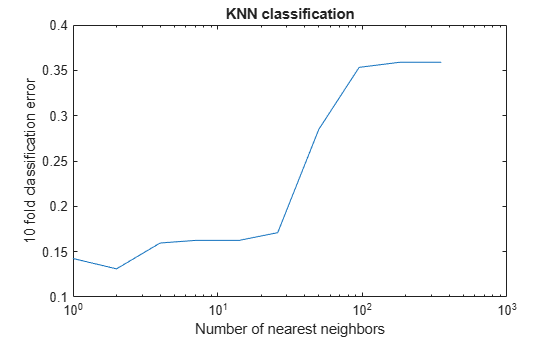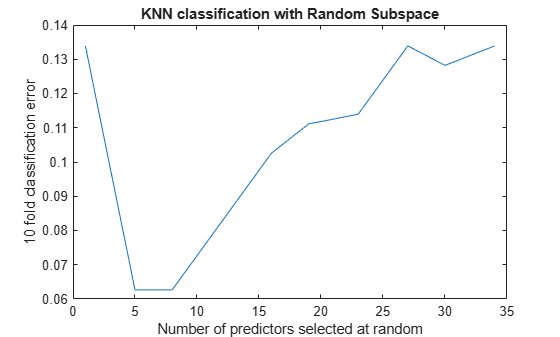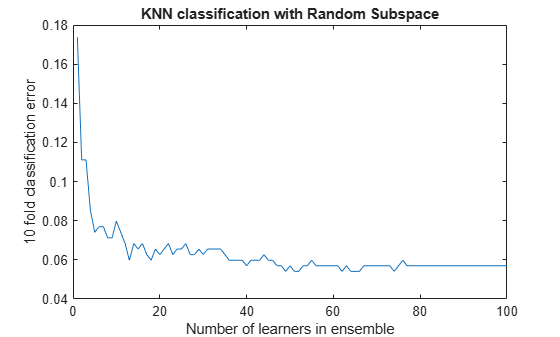# Random Subspace Classification

This example shows how to use a random subspace ensemble to increase the accuracy of classification. It also shows how to use cross validation to determine good parameters for both the weak learner template and the ensemble.

### Load the data

Load the `ionosphere` data. This data has 351 binary responses to 34 predictors.

```load ionosphere; [N,D] = size(X)```
```N = 351 ```
```D = 34 ```
`resp = unique(Y)`
```resp = 2x1 cell {'b'} {'g'} ```

### Choose the number of nearest neighbors

Find a good choice for `k`, the number of nearest neighbors in the classifier, by cross validation. Choose the number of neighbors approximately evenly spaced on a logarithmic scale.

```rng(8000,'twister') % for reproducibility K = round(logspace(0,log10(N),10)); % number of neighbors cvloss = zeros(numel(K),1); for k=1:numel(K) knn = fitcknn(X,Y,... 'NumNeighbors',K(k),'CrossVal','On'); cvloss(k) = kfoldLoss(knn); end figure; % Plot the accuracy versus k semilogx(K,cvloss); xlabel('Number of nearest neighbors'); ylabel('10 fold classification error'); title('k-NN classification');```The lowest cross-validation error occurs for `k = 2`.

### Create the ensembles

Create ensembles for `2`-nearest neighbor classification with various numbers of dimensions, and examine the cross-validated loss of the resulting ensembles.

This step takes a long time. To keep track of the progress, print a message as each dimension finishes.

```NPredToSample = round(linspace(1,D,10)); % linear spacing of dimensions cvloss = zeros(numel(NPredToSample),1); learner = templateKNN('NumNeighbors',2); for npred=1:numel(NPredToSample) subspace = fitcensemble(X,Y,'Method','Subspace','Learners',learner, ... 'NPredToSample',NPredToSample(npred),'CrossVal','On'); cvloss(npred) = kfoldLoss(subspace); fprintf('Random Subspace %i done.\n',npred); end```
```Random Subspace 1 done. Random Subspace 2 done. Random Subspace 3 done. Random Subspace 4 done. Random Subspace 5 done. Random Subspace 6 done. Random Subspace 7 done. Random Subspace 8 done. Random Subspace 9 done. Random Subspace 10 done. ```
```figure; % plot the accuracy versus dimension plot(NPredToSample,cvloss); xlabel('Number of predictors selected at random'); ylabel('10 fold classification error'); title('k-NN classification with Random Subspace');```The ensembles that use five and eight predictors per learner have the lowest cross-validated error. The error rate for these ensembles is about 0.06, while the other ensembles have cross-validated error rates that are approximately 0.1 or more.

### Find a good ensemble size

Find the smallest number of learners in the ensemble that still give good classification.

```ens = fitcensemble(X,Y,'Method','Subspace','Learners',learner, ... 'NPredToSample',5,'CrossVal','on'); figure; % Plot the accuracy versus number in ensemble plot(kfoldLoss(ens,'Mode','Cumulative')) xlabel('Number of learners in ensemble'); ylabel('10 fold classification error'); title('k-NN classification with Random Subspace');```There seems to be no advantage in an ensemble with more than 50 or so learners. It is possible that 25 learners gives good predictions.

### Create a final ensemble

Construct a final ensemble with 50 learners. Compact the ensemble and see if the compacted version saves an appreciable amount of memory.

```ens = fitcensemble(X,Y,'Method','Subspace','NumLearningCycles',50,... 'Learners',learner,'NPredToSample',5); cens = compact(ens); s1 = whos('ens'); s2 = whos('cens'); [s1.bytes s2.bytes] % si.bytes = size in bytes```
```ans = 1×2 1748675 1518820 ```

The compact ensemble is about 10% smaller than the full ensemble. Both give the same predictions.